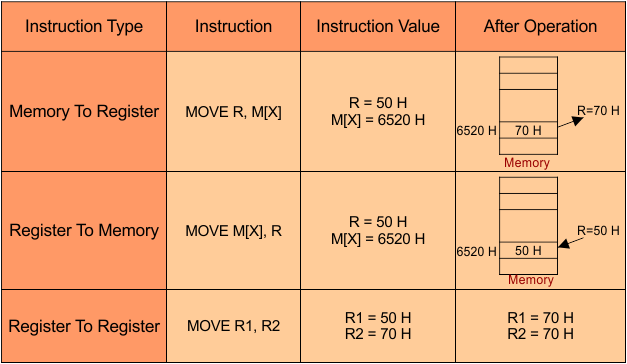Select Page

Computer Organization

Memory Organization
Interrupt
DMA Controller

# Data Transfer Instructions

Data transfer instructions basically include the instructions that are used to transfer data from one place to another (i.e. within the system or system to I/O device).  These instructions are also known as copy instructions.

## Data Transfer Methods

The data movement cases are given below

• Memory to Register
• Register to Memory
• Register to Register

## Data Transfer Instructions Table

Let Understand the data transfer instructions table## Types of Data Transfer Instructions

Some mostly using data transfer instructions are given below

• Symbolic (Mnemonics) of LOAD instruction is LD
• Example of LOAD instruction is LD AX, BX

Explanation: Load from the source to the destination. As in a above example, AX is Destination and BX is Source. Before the LOAD instruction execution AX=3,and BX=5 and after Execution of LOAD Instruction AX= 5 , BX=5.

### 2. STORE

• Symbolic (Mnemonics) of STORE instruction is ST
• Example of LOAD instruction is ST AX, BX

Explanation: It means, store the value from the source and place it in the destination. As in a above example, AX is Destination and BX is Source. So,  After STORE instruction execution AX=BX.

### 3. MOVE

• Symbolic (Mnemonics) of MOVE instruction is MOV
• Example of MOVE instruction is MOV AX, BX

Explanation: It means, Move the value from the source and place it in the destination. As AX is Destination and BX is Source. So, After MOVE instruction execution AX=BX.

### 4. MOVE IMMEDIATE

• Symbolic (Mnemonics) of MOVE IMMEDIATE instruction is MVI
• Example of MOVE IMMEDIATE instruction is MVI AX, BX

Explanation: MVI AX, BX means that BX will be copied to the register AX. If you want to take instant input from the user then  MVI instruction is used. It is two byte instruction in which one byte contains an 8-bit op-code and other byte contains 8-bit operand (Data) and two machine cycle with seven T series.

### 5. EXCHANGE

• Symbolic (Mnemonics) of EXCHANGE instruction is XCH
• Example of EXCHANGE instruction is XCH AX, BX

Explanation: Exchange Interchange the value of source and destination in above example. If AX=3, BX=5 then after exchange execution AX= 5 , BX=3.

### 6. INPUT

• Symbolic (Mnemonics) of INPUT instruction is INPUT
• Example of INPUT instruction is INPUT AX, BX

Explanation: Content of input port is read and loaded into Accumulator Register through 8bit Bus. In above diagram,  A word is read from 00FFH address port to AX register.

### 7. OUTPUT

• Symbolic (Mnemonics) of OUTPUT instruction is OUT
• Example of OUTPUT instruction is OUT AX, 00FFH

Explanation: This instruction shows the result of AX register in above example.

### 8. PUSH

• Symbolic (Mnemonics) of PUSH instruction is PUSH
• Example of PUSH instruction is PUSH a

Explanation: It means, Push data to stack Memory.

### 8. POP

• Symbolic (Mnemonics) of POP instruction is POP
• Example of POP instruction is POP a

Explanation: It means, POP data from stack Memory.

Note: These  All above Instructions do not affect flag register; carry register, sign, and parity flag.

Help Other’s By Sharing…